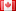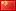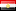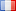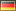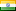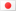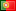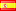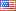Saturating grade decorative papers are generally impregnated with thermoset resins (melamine, polyester and phenolic resins) and are thermally fused to special backers or substrates depending on the final product. These papers are used to manufacturer HPL (High Pressure Laminate), TFM (Thermally Fused Melamine), Laminate Flooring, CPL (Continuously Pressed Laminate), Thermo Fused Laminates and Post Impregnated Foils. There are many other uses that are too numerous to list.

Un-impregnated papers sold by the paper manufacturers and or printers and are generally sold by weight. However the end products are almost always sold by area, length or piece so the need to convert from weight (mass) to other units of measure is essential.

The following calculators were created to make these conversions easy and flexible, allowing mixed entries between metric and imperial units of measure. Click on the blue title bar to navigate to the desired calculator.

Basis weight, grammage and coverage conversions

This calculator can convert between any one of the four following units:

• Grammage (g / m2) - the number of grams in one square meter of paper
• Basis Weight (lbs / 3,000 ft2) - the number of pounds in 3,000 square feet of paper
• Coverage (metric) - the number of square meters (m2) that one kilogram of paper covers
• Coverage (imperial) - the number of square feet (ft2) that one pound of paper covers

Cost conversions between area and weight

This calculator can convert costs between area or weight if you provide the following information:

• Grammage (g / m2or basis weight (lbs / 3,000 ft2) *required
• And one of the four following units
• Cost by area in either dollars \$ / square meter (m2) or dollars \$ / square foot (ft2)
• Cost in weight in either dollars \$ / kilogram (kg) or dollars \$ / pound (lb)

New option now available - a second calculator can be opened that allows you to convert the cost to other currencies!

Length of a roll by diameter and thickness of the paper

This calculator can calculate the length of roll of paper if you provide the following information:

• Diameter of the roll in millimeters (mm) or inches (in)
• Outside diameter (OD) of the core in millimeters (mm) or inches (in)
• Thickness of the paper in microns or mils (1/1,000 inch)

Length of a roll by width and weight of the roll

This calculator can calculate the length of roll of paper if you provide the following information:

• Grammage (g / m2or basis weight (lbs / 3,000 ft2)
• Width of the paper in millimeters (mm) or inches (in)
• Weight of the roll of paper in kilograms (kg) or pounds (lbs)

Project calculations by area or weight

This calculator can convert between area and weight with the following information:

• Grammage (g / m2or basis weight (lbs / 3,000 ft2) *required
• And one of the following units
• Area of the project in square meters (m2) or square Feet (ft2)
• Weight of paper in kilograms (kg) or pounds (lbs)

Project calculations by faces or weight

This calculator converts between the number of faces and weight required for a project with the following information:

• Grammage (g / m2or basis weight (lbs / 3,000 ft2) *required
• Dimensions (width and length) of the faces in millimeters (mm) or inches (in) *required
• And one of the following units
• Number of faces for the project
• Weight of the paper in kilograms (kg) or pounds (lbs)

Weight of a roll by width and length of the roll

This calculator can find the weight of roll of paper if you provide the following information:

• Grammage (g / m2or basis weight (lbs / 3,000 ft2)
• Width of the paper in millimeters (mm) or inches (in)
• Length of the roll of paper in meters (m) or feet (ft)

DÉCOR PLANit
Created and maintained by Robert J Knothe